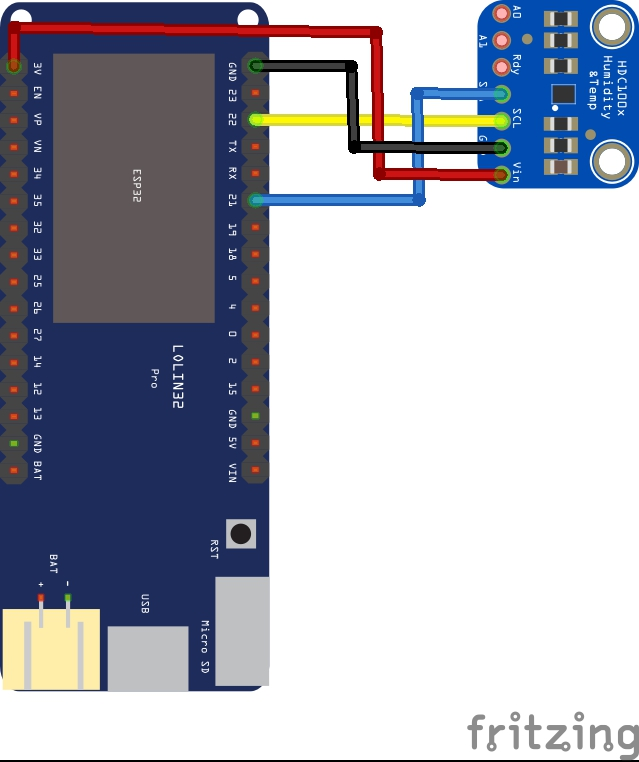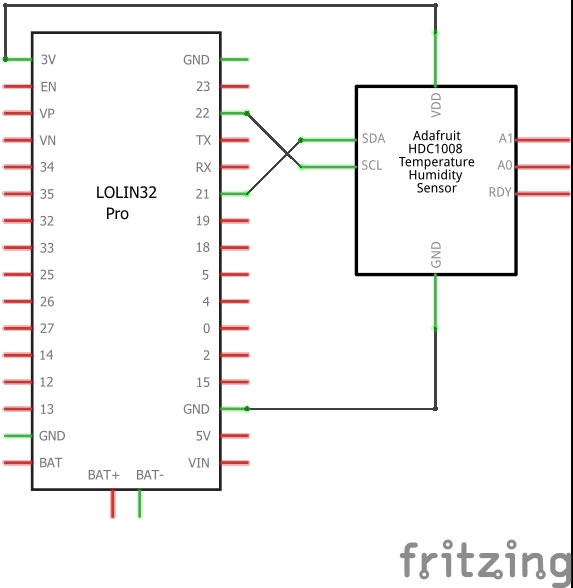Home Code A HDC1008 digital humidity sensor Micropython example on an ESP32

# A HDC1008 digital humidity sensor Micropython example on an ESP32

In this example we connect a HDC1008 digital humidity sensor to an ESP32 running Micropython

The HDC1008 is a digital humidity sensor with integrated temperature sensor that provides excellent measurement accuracy at very low power. The device measures humidity based on a novel capacitive sensor. The humidity and temperature sensors are factory calibrated. The innovative WLCSP (Wafer Level Chip Scale Package) simplifies board design with the use of an ultra-compact package. The sensing element of the HDC1008 is placed on the bottom part of the device, which makes the HDC1008 more robust against dirt, dust, and other environmental contaminants. The HDC1008 is functional within the full –40°C to +125°C temperature range.

## Features

• Relative Humidity (RH) Operating Range 0% to
100%
• 14 Bit Measurement Resolution
• Relative Humidity Accuracy ±4%
• Temperature Accuracy ±0.2 °C
• 200 nA Sleep Mode Current
• Average Supply Current:
• 820 nA @ 1sps, 11 bit RH Measurement
• 1.2 µA @ 1sps, 11 bit RH and Temperature
Measurement
• Supply Voltage 3 V to 5 V
• Tiny 2 mm × 1.6 mm Device Footprint
• I2C Interface

Parts List

### Connection

An easy module to connect to an ESP32, SCL is 22 and SDA is 21 on the Wemos board I used, you can see this is the schematic below### Code

You can use any method to upload files or an IDE for development.

The following is based on a github library – https://github.com/kfricke/micropython-hdc1008. The first part of this is the library which I upload to my ESP32

This is the library called hdc1008.py

[codesyntax lang=”python”]

```from machine import I2C
from utime import sleep_ms

REG_TEMP = const(0x00)
REG_HUMI = const(0x01)
REG_CFG = const(0x02)
REG_SER_ID1 = const(0xfb)
REG_SER_ID2 = const(0xfc)
REG_SER_ID3 = const(0xfd)

CFG_RST = const(0b10000000)
CFG_HEAT_ON = const(0b100000)
CFG_HEAT_OFF = const(0b0)
CFG_MODE_SINGLE = const(0b0)
CFG_MODE_BOTH = const(0b10000)
CFG_BTST = const(0b1000)
CFG_TEMP_14BIT = const(0b0)
CFG_TEMP_11BIT = const(0b100)
CFG_HUMI_14BIT = const(0b0)
CFG_HUMI_11BIT = const(0b1)
CFG_HUMI_8BIT = const(0b10)

RES_8_BIT  = const(1)
RES_11_BIT = const(2)
RES_14_BIT = const(3)

class HDC1008(object):
if i2c == None or i2c.__class__ != I2C:
raise ValueError('I2C object needed as argument!')
self._i2c = i2c
self._heater = heater
self._tmp = bytearray(4)
# Read the sensor serial ID
self.serial = 0
self._send_byte(REG_SER_ID1)
self._recv(self._tmp, 2)
self.serial += (self._tmp << 32) + (self._tmp << 24)
self._send_byte(REG_SER_ID2)
self._recv(self._tmp, 2)
self.serial += (self._tmp << 16) + (self._tmp << 8)
self._send_byte(REG_SER_ID3)
self._recv(self._tmp, 2)
self.serial += self._tmp

def _send_byte(self, b):
self._tmp = b

def _send_bytes(self, b):

def _recv(self, buf, c):

def _config(self, reg=None):
if reg is None:
self._send_byte(REG_CFG)
self._recv(self._tmp, 2)
return self._tmp[0:2]
else:
if self._heater:
reg = reg | CFG_HEAT_ON
self._tmp = REG_CFG
self._tmp = reg
self._tmp = 0
self._send_bytes(self._tmp[0:3])

def heater(self, s=None):
if s is not None:
if s.__class__ != bool:
raise ValueError('Heater state must be a boolean value or None!')
else:
self._heater = s
else:
return self._heater

def battery_low(self):
# one read command needed to have the config register defined, where
# we can read this flag from
self._raw_temp(CFG_TEMP_11BIT)
return (self._config() & CFG_BTST) == CFG_BTST

def reset(self):
self._tmp = REG_CFG
self._tmp = CFG_RST
self._tmp = 0
self._send_bytes(self._tmp[0:3])
sleep_ms(20)

def _raw_temp(self, acc):
self._config(CFG_MODE_SINGLE | acc)
self._send_byte(REG_TEMP)
sleep_ms(15)
self._recv(self._tmp, 2)
return self._tmp + (self._tmp << 8)

def temp(self, acc=CFG_TEMP_14BIT):
return (self._raw_temp(acc) / 65536) * 165 - 40

def _raw_humi(self, acc):
self._config(CFG_MODE_SINGLE | acc)
self._send_byte(REG_HUMI)
sleep_ms(13)
self._recv(self._tmp, 2)
return self._tmp + (self._tmp << 8)

def humi(self, acc=CFG_HUMI_14BIT):
return (self._raw_humi(acc) / 65536.0) * 100.0

def _raw_temp_humi(self, t_acc, h_acc):
self._config(CFG_MODE_BOTH | t_acc | h_acc)
self._send_byte(REG_TEMP)
sleep_ms(20)
self._recv(self._tmp, 4)
return (self._tmp + (self._tmp << 8), self._tmp + (self._tmp << 8))

def temp_humi(self, t_acc=CFG_TEMP_14BIT, h_acc=CFG_HUMI_14BIT):
(raw_temp, raw_humi) = self._raw_temp_humi(t_acc, h_acc)
return (((raw_temp / 65536) * 165) - 40, (raw_humi / 65536) * 100.0)
```

[/codesyntax]

I upload this file to my ESP32 and now in main.py I copy the following code in

[codesyntax lang=”python”]

```import machine
try:
import esp
esp.osdebug(None)
i2c = machine.I2C(sda=machine.Pin(4), scl=machine.Pin(5))
except:
pass
try:
import pyb
i2c = machine.I2C(sda=machine.Pin('X10'), scl=machine.Pin('X9'), freq=400000)
except:
pass
import utime
from hdc1008 import HDC1008

hdc = HDC1008(i2c)
hdc.reset()
hdc.heater(False)
print("Sensor ID: %s" % (hex(hdc.serial)))

print("Temperature (degree celsius): %.2f" % (hdc.temp()))
print("Relative humidity (percent):  %.2f" % (hdc.humi()))
print("Both sensors read at once:    %.2f  %.2f" % hdc.temp_humi())
print("Battery low: %s" % (hdc.battery_low()))

print("Reading sensors 10 times using idle sleeping...")
for i in range(10):
utime.sleep_ms(100)```

[/codesyntax]

### Testing

Open up the REPL window. Here is what I saw in uPyCraft

…….
Sensor ID: 0x224f67673
Reading sensors 10 times using idle sleeping…
Temperature (degree celsius): 22.99
Relative humidity (percent): 33.60
Both sensors read at once: 23.01 33.60
Battery low: False
Temperature (degree celsius): 23.01
Relative humidity (percent): 33.60
Both sensors read at once: 22.99 33.60
Battery low: False
Temperature (degree celsius): 22.99
Relative humidity (percent): 33.50
Both sensors read at once: 22.99 33.50
Battery low: False
Temperature (degree celsius): 22.99
Relative humidity (percent): 33.50
Both sensors read at once: 22.99 33.50
Battery low: False
Temperature (degree celsius): 22.97
Relative humidity (percent): 33.50
Both sensors read at once: 22.96 33.50
Battery low: False